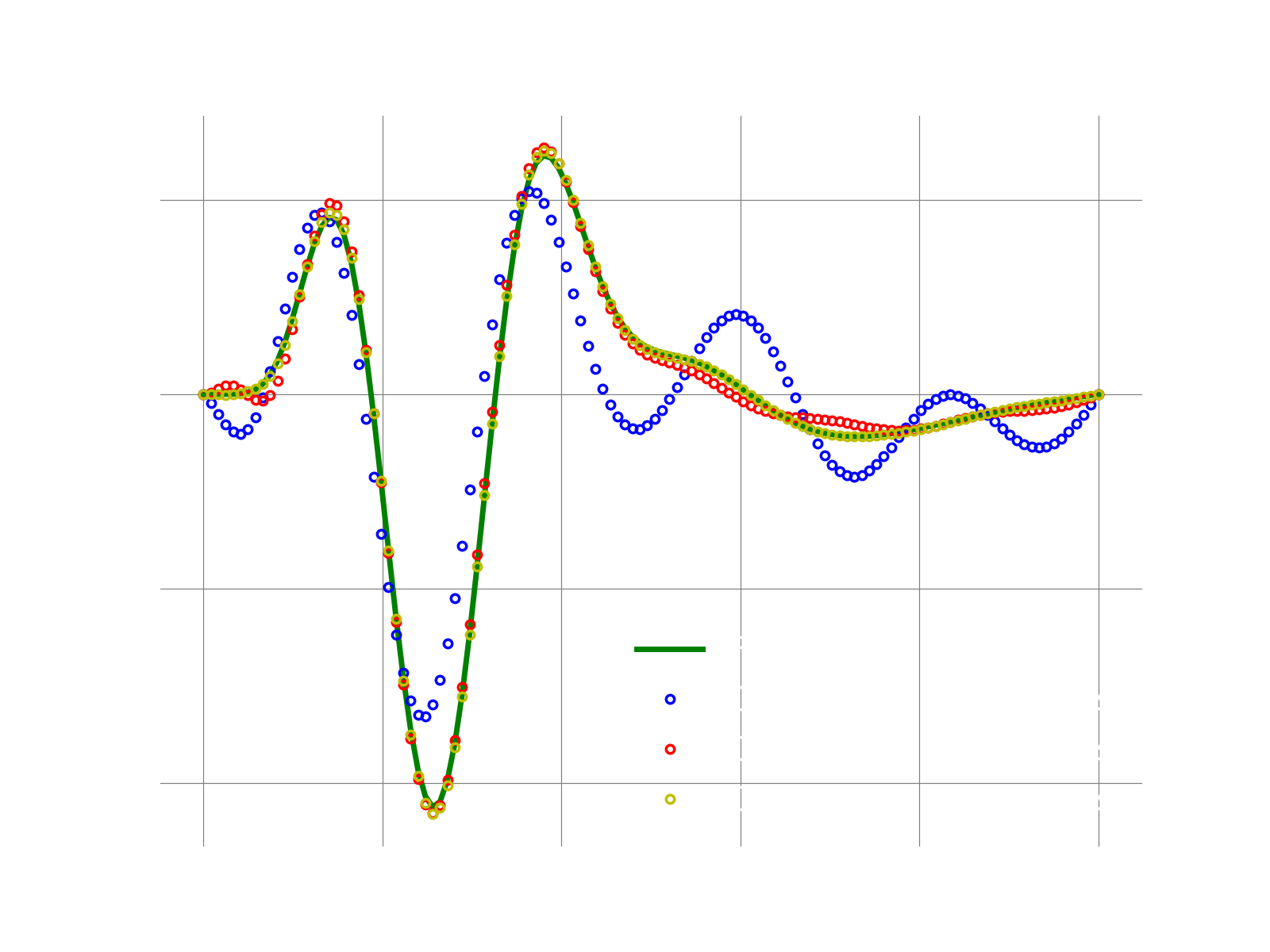# 1D adv-diff: Galerkin with POD modes

## Overview

We cover these three typical steps needed for a ROM:

1. generate of snapshots using the full-order model (FOM)
2. compute the basis: here we demonstrate the use of POD modes
3. execute the ROM: here we leverage the GALERKIN ROM to demonstrate a reproductive test, i.e., we run the ROM using the same physical coefficients, b.c., etc. A predictive run is demonstrated in a different demo.

## FOM Equations

The governing equations for this problem are:

where , the field is , the advection velocity is fixed at , the spatial coordinate is and the domain is . We use homogeneous BC. Note that a class approximating the FOM operators via finite-differences is implemented here.

## Main function

The main function of the demo is the following:

logger.initialize(logger.logto.terminal)
logger.setVerbosity([logger.loglevel.info])

# create fom object

# the final time to integrate to
finalTime = .05

#--- 1. FOM ---#
fomTimeStepSize  = 1e-5
fomNumberOfSteps = int(finalTime/fomTimeStepSize)
sampleEvery      = 200
[fomFinalState, snapshots] = doFom(fomObj, fomTimeStepSize, fomNumberOfSteps, sampleEvery)

#--- 2. POD ---#
modes = computePodModes(snapshots)

#--- 3. GALERKIN ROM ---#
romTimeStepSize  = 3e-4
romNumberOfSteps = int(finalTime/romTimeStepSize)
# run with various number of modes
romSizes = [2,4,6]
approximations = {}
for romSize in romSizes:
currentSolution = runGalerkin(fomObj, romTimeStepSize,
romNumberOfSteps,
modes[:,:romSize])
approximations[romSize] = currentSolution

# compute l2-error between fom and approximate state
fomNorm = linalg.norm(fomFinalState)
err = linalg.norm(fomFinalState-currentSolution)
print("With {} modes, final relative l2 error: {}".format(romSize, err/fomNorm))

### 1. Run FOM and collect snapshots

def doFom(fom, dt, nsteps, saveFreq):
u = fom.u0.copy()
U = [u]
f = fom.createVelocity()
for i in range(1,nsteps+1):
# query rhs of discretized system
fom.velocity(u, i*dt, f)
# simple Euler forward
u = u + dt*f
if i % saveFreq == 0:
U.append(u)
Usolns = np.array(U)
return [u, Usolns.T]

### 2. Compute POD modes

print("SVD on matrix: ", snapshots.shape)
U,S,VT = np.linalg.svd(snapshots)
return U

### 3. Construct and run ROM

def runGalerkin(fomObj, dt, nsteps, modes):
# auxiliary class to use in the solve below
# to monitor the rom state during time stepping
class RomStateObserver:
def __call__(self, timeStep, time, state): pass

# find out number of modes wanted
romSize = modes.shape

# create a linear decoder, passing only the desired number of modes
# this will make a deep copy of the modes
linearDecoder = rom.Decoder(modes)

# fom reference state: here it is zero
fomReferenceState = np.zeros(fomObj.nGrid)

# create ROM state by projecting the fom initial condition
fomInitialState = fomObj.u0.copy()
romState = np.dot(modes.T, fomInitialState)

# create problem
scheme = ode.stepscheme.ForwardEuler
problem = rom.galerkin.DefaultExplicitProblem(scheme, fomObj, linearDecoder, romState, fomReferenceState)

# create object to monitor the romState at every iteration
myObs = RomStateObserver()
# solve problem
ode.advance_n_steps_and_observe(problem, romState, 0., dt, nsteps, myObs)

# after we are done, use the reconstructor object to reconstruct the fom state
# get the reconstructor object: this allows to map romState to fomState
fomRecon = problem.fomStateReconstructor()
return fomRecon(romState)

## Results

If everything works fine, the following plot shows the result. We see that for this toy example, the full solution is recovered very well with Galerkin with just a few POD modes.